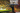# Binary Tree - Level Order Traversal

November 19, 2020## Problem Statement

Given a Binary tree, print out nodes in level order traversal from left to right.

``````        50
/    \
80      30
/    \      \
20     40      10

# Output
# 50 80 30 20 40 10``````

## Approach-1

Use BFS (Breadth First Search) algorithm. Since, it reaches out to nodes first that are immediate neighbours.

Idea is to take a queue, keep accumulating queue for each child.

``````void bfs(Node node) {
Queue<Node> q = new Queue();

while (!q.isEmpty()) {
Node n = q.pop();
print(n);

}
}``````

The `Complexity` is `O(n)` as we are visiting each nodes only once.

## Approach-2

You can prepare a list of nodes at each level. We will also use DFS (Depth First Search) algorithm here.

``````void levelOrder(Node node, List<List<Node>> list, int level) {
if (node == null)
return;
List<Node> levelList = list.get(level);
if (levelList == null) {
levelList = new ArrayList();
}

levelOrder(node.left, list, level+1);
levelOrder(node.right, list, level+1);
}

List<List<Node>> list = new ArrayList();
levelOrder(root, list, 0);``````

After this, we can print the list.

The `Complexity` is `O(n)` as we are visiting each nodes only once.